Contemporary Mathematics

# 10.5Tessellations

Contemporary Mathematics10.5 Tessellations

Figure 10.77 Penrose tiling represents one type of tessellation. (credit: "Penrose Tiling" by Inductiveload/Wikimedia Commons, Public Domain)

### Learning Objectives

After completing this section, you should be able to:

1. Apply translations, rotations, and reflections.
2. Determine if a shape tessellates.

The illustration shown above (Figure 10.77) is an unusual pattern called a Penrose tiling. Notice that there are two types of shapes used throughout the pattern: smaller green parallelograms and larger blue parallelograms. What's interesting about this design is that although it uses only two shapes over and over, there is no repeating pattern.

In this section, we will focus on patterns that do repeat. Repeated patterns are found in architecture, fabric, floor tiles, wall patterns, rug patterns, and many unexpected places as well. It may be a simple hexagon-shaped floor tile, or a complex pattern composed of several different motifs. These two-dimensional designs are called regular (or periodic) tessellations. There are countless designs that may be classified as regular tessellations, and they all have one thing in common—their patterns repeat and cover the plane.

We will explore how tessellations are created and experiment with making some of our own as well. The topic of tessellations belongs to a field in mathematics called transformational geometry, which is a study of the ways objects can be moved while retaining the same shape and size. These movements are termed rigid motions and symmetries.

### Who Knew?

#### M. C. Escher

A good place to start the study of tessellations is with the work of M. C. Escher. The Dutch graphic artist was famous for the dimensional illusions he created in his woodcuts and lithographs, and that theme is carried out in many of his tessellations as well. Escher became obsessed with the idea of the “regular division of the plane.” He sought ways to divide the plane with shapes that would fit snugly next to each other with no gaps or overlaps, represent beautiful patterns, and could be repeated infinitely to fill the plane. He experimented with practically every geometric shape imaginable and found the ones that would produce a regular division of the plane. The idea is similar to dividing a number by one of its factors. When a number divides another number evenly, there are no remainders, like there are no gaps when a shape divides or fills the plane.

Escher went far beyond geometric shapes, beyond triangles and polygons, beyond irregular polygons, and used other shapes like figures, faces, animals, fish, and practically any type of object to achieve his goal; and he did achieve it, beautifully, and left it for the ages to appreciate.

### Tessellation Properties and Transformations

A regular tessellation means that the pattern is made up of congruent regular polygons, same size and shape, including some type of movement; that is, some type of transformation or symmetry. Here we consider the rigid motions of translations, rotations, reflections, or glide reflections. A plane of tessellations has the following properties:

• Patterns are repeated and fill the plane.
• There are no gaps or overlaps. Shapes must fit together perfectly. (It was Escher who determined that a proper tessellation could have no gaps and no overlaps.)
• Shapes are combined using a transformation.
• All the shapes are joined at a vertex. In other words, if you were to draw a circle around a vertex, it would include a corner of each shape touching at that vertex.
• For a tessellation of regular congruent polygons, the sum of the measures of the interior angles that meet at a vertex equals $360∘.360∘.$

In Figure 10.78, the tessellation is made up of squares. There are four squares meeting at a vertex. An interior angle of a square is $90∘90∘$ and the sum of four interior angles is $360∘.360∘.$ In Figure 10.79, the tessellation is made up of regular hexagons. There are three hexagons meeting at each vertex. The interior angle of a hexagon is $120∘,120∘,$ and the sum of three interior angles is $360∘.360∘.$ Both tessellations will fill the plane, there are no gaps, the sum of the interior angle meeting at the vertex is $360∘,360∘,$ and both are achieved by translation transformations. These tessellations work because all the properties of a tessellation are present.

Figure 10.78 Tessellation – Squares
Figure 10.79 Tessellation – Hexagons

The movements or rigid motions of the shapes that define tessellations are classified as translations, rotations, reflections, or glide reflections. Let’s first define these movements and then look at some examples showing how these transformations are revealed.

#### Translation

A translation is a movement that shifts the shape vertically, horizontally, or on the diagonal. Consider the trapezoid $ABCDABCD$ in Figure 10.80. We have translated it 3 units to the right and 3 units up. That means every corner is moved by the number of units and in the direction specified. Mathematicians will indicate this movement with a vector, an arrow that is drawn to illustrate the criteria and the magnitude of the translation. The location of the translated trapezoid is marked with the vertices, $A′B′C′D′,A′B′C′D′,$ but it is still the exact same shape and size as the original trapezoid $ABCDABCD$.

Figure 10.80 Translation

### Example 10.34

#### Creating a Translation

Suppose you have a hexagon on a grid as in Figure 10.81. Translate the hexagon 5 units to the right and 3 units up.

Figure 10.81

1.
Translate the hexagon with points $A',$ $B',$ $C',$ $D',$ $E',$ $F'$ 6 units down.

#### Rotation

The rotation transformation occurs when you rotate a shape about a point and at a predetermined angle. In Figure 10.83, the triangle is rotated around the rotation point by $90∘,90∘,$ and then translated 7 units up and 4 units over to the right. That means that each corner is translated to the new location by the same number of units and in the same direction.

Figure 10.83 Rotation

We can see that $ΔAΔA$ is mapped to $ΔA′ΔA′$ by a rotation of $90∘90∘$ up and to the right. If rotated again by $90∘90∘$, the triangle would be upside down.

### Example 10.35

#### Applying a Rotation

Figure 10.84 illustrates a tessellation begun with an equilateral triangle. Explain how this pattern is produced.

Figure 10.84

1.
Starting with the triangle in the figure shown, explain how the pattern on the right was achieved.

#### Reflection

A reflection is the third transformation. A shape is reflected about a line and the new shape becomes a mirror image. You can reflect the shape vertically, horizontally, or on the diagonal. There are two shapes in Figure 10.85. The quadrilateral is reflected horizontally; the arrow shape is reflected vertically.

Figure 10.85 Reflection

#### Glide Reflection

The glide reflection is the fourth transformation. It is a combination of a reflection and a translation. This can occur by first reflecting the shape and then gliding or translating it to its new location, or by translating first and then reflecting. The example in Figure 10.86 shows a trapezoid, which is reflected over the dashed line, so it appears upside down. Then, we shifted the shape horizontally by 6 units to the right. Whether we use the glide first or the reflection first, the end result is the same in most cases. However, the tessellation shown in the next example can only be achieved by a reflection first and then a translation.

Figure 10.86 Glide Reflection

### Example 10.36

#### Applying the Glide Reflection

An obtuse triangle is reflected about the dashed line, and the two shapes are joined together. How does the tessellation shown in Figure 10.87 materialize?

Figure 10.87

1.
Explain how this tessellation of equilateral triangles could be produced.

### Example 10.37

#### Applying More Than One Tessellation

Show how this tessellation (Figure 10.88) can be achieved.

Figure 10.88

1.
How does this tessellation of the squares come about?

### Interior Angles

The sum of the interior angles of a tessellation is $360∘360∘$. In Figure 10.89, the tessellation is made of six triangles formed into the shape of a hexagon. Each angle inside a triangle equals $60∘60∘$, and the six vertices meet the sum of those interior angles, $6(60°)=360°6(60°)=360°$.

Figure 10.89 Interior Angles at the Vertex of Triangles

In Figure 10.90, the tessellation is made up of trapezoids, such that two of the interior angles of each trapezoid equals $75°75°$ and the other two angles equal $105°105°$. Thus, the sum of the interior angles where the vertices of four trapezoids meet equals $105°+75°+75°+105°=360°105°+75°+75°+105°=360°$.

Figure 10.90 Interior Angles at the Vertex of Trapezoids

These tessellations illustrate the property that the shapes meet at a vertex where the interior angles sum to $360°360°$.

### Tessellating Shapes

We might think that all regular polygons will tessellate the plane by themselves. We have seen that squares do and hexagons do. The pattern of squares in Figure 10.91 is a translation of the shape horizontally and vertically. The hexagonal pattern in Figure 10.92, is translated horizontally, and then on the diagonal, either to the right or to the left. This particular pattern can also be formed by rotations. Both tessellations are made up of congruent shapes and each shape fits in perfectly as the pattern repeats.

Figure 10.91 Translation Horizontally and Vertically
Figure 10.92 Translation Horizontally and Slide Diagonally

We have also seen that equilateral triangles will tessellate the plane without gaps or overlaps, as shown in Figure 10.93. The pattern is made by a reflection and a translation. The darker side is the face of the triangle and the lighter side is the back of the triangle, shown by the reflection. Each triangle is reflected and then translated on the diagonal.

Figure 10.93 Reflection and Glide Translation

Escher experimented with all regular polygons and found that only the ones mentioned, the equilateral triangle, the square, and the hexagon, will tessellate the plane by themselves. Let’s try a few other regular polygons to observe what Escher found.

### Example 10.38

#### Tessellating the Plane

Do regular pentagons tessellate the plane by themselves (Figure 10.94)?

Figure 10.94

1.
Do regular heptagons tessellate the plane by themselves?

### Example 10.39

#### Tessellating Octagons

Do regular octagons tessellate the plane by themselves (Figure 10.95)?

Figure 10.95

1.
Do regular dodecagons (12-sided regular polygons) tessellate the plane by themselves?

Just because regular pentagons do not tessellate the plane by themselves does not mean that there are no pentagons that tessellate the plane, as we see in Figure 10.96.

Figure 10.96 Tessellation of Pentagons

Another example of an irregular polygon that tessellates the plane is by using the obtuse irregular triangle from a previous example. What transformations should be performed to produce the tessellation shown in Figure 10.97?

Figure 10.97 Tessellating with Obtuse Irregular Triangles

First, the triangle is reflected over the tip at point $AA$, and then translated to the right and joined with the original triangle to form a parallelogram. The parallelogram is then translated on the diagonal and to the right and to the left.

#### Naming

A tessellation of squares is named by choosing a vertex and then counting the number of sides of each shape touching the vertex. Each square in the tessellation shown in Figure 10.98 has four sides, so starting with square $AA$, the first number is 4, moving around counterclockwise to the next square meeting the vertex, square $BB$, we have another 4, square $CC$ adds another 4, and finally square $DD$ adds a fourth 4. So, we would name this tessellation a 4.4.4.4.

The hexagon tessellation, shown in Figure 10.99 has six sides to the shape and three hexagons meet at the vertex. Thus, we would name this a 6.6.6. The triangle tessellation, shown in Figure 10.100 has six triangles meeting the vertex. Each triangle has three sides. Thus, we name this a 3.3.3.3.3.3.

Figure 10.98 4.4.4.4
Figure 10.99 6.6.6
Figure 10.100 3.3.3.3.3.3

### Example 10.40

Create a tessellation using two colors and two shapes.

1.
Create a tessellation using polygons, regular or irregular.

30.
What are the properties of repeated patterns that let them be classified as tessellations?
31.
Explain how the using the transformation of a translation is applied to the movement of this shape starting with point $A$.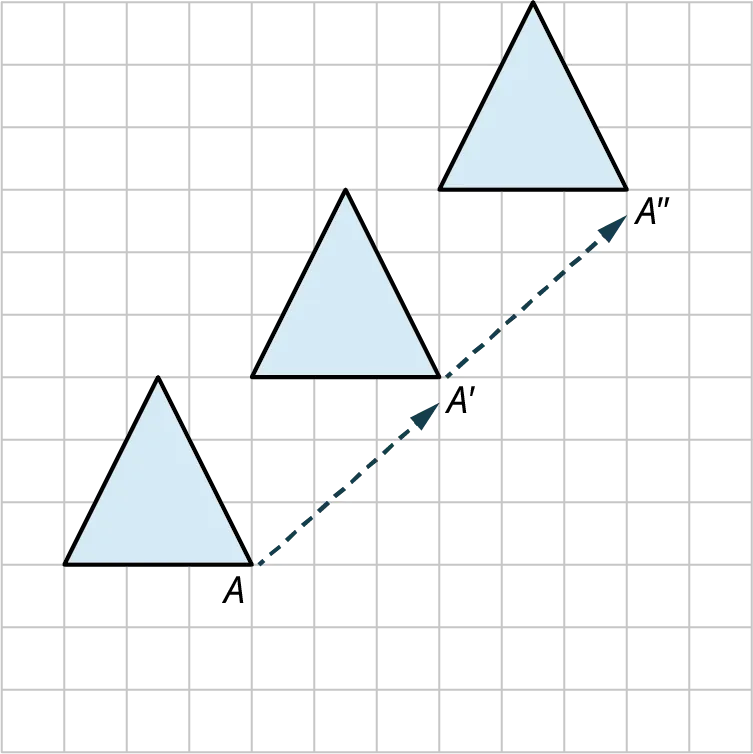32.
Starting with the triangle with vertex $B$, describe how the transformation in this drawing is achieved.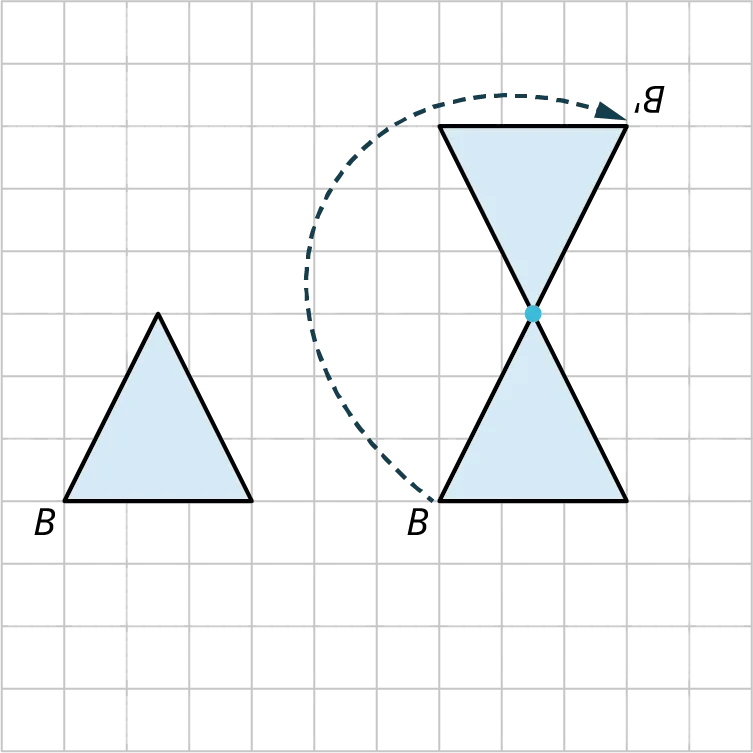33.
Starting with a triangle with a darker face and a lighter back, describe how this pattern came about.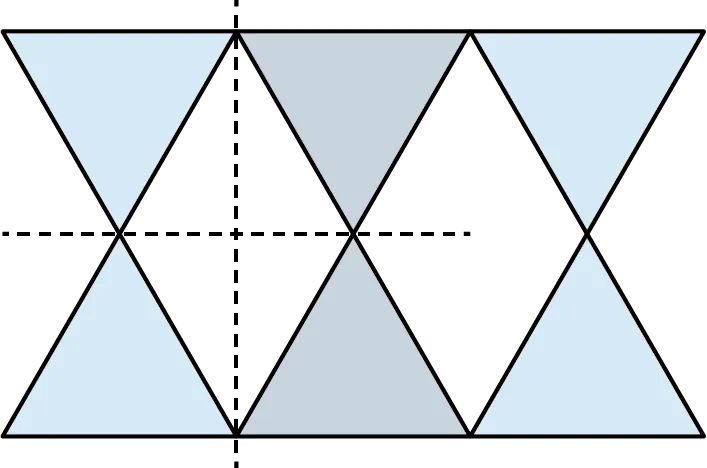34.
Name the tessellation in the figure shown.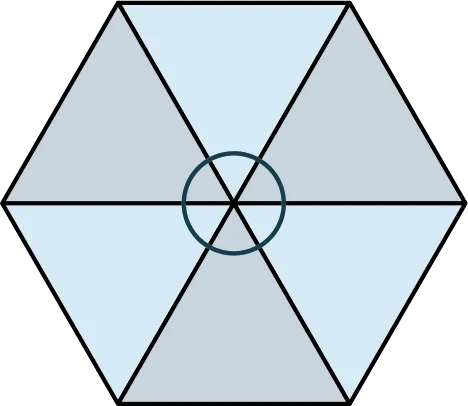### Section 10.5 Exercises

1 .
What type of movements are used to change the orientation and placement of a shape?
2 .
What is the name of the motion that renders a shape upside down?
3 .
What do we call the motion that moves a shape to the right or left or on the diagonal?
4 .
If you are going to tessellate the plane with a regular polygon, what is the sum of the interior angles that surround a vertex?
5 .
Does a regular heptagon tesselate the plane by itself?
6 .
What are the only regular polygons that will tessellate the plane by themselves?
7 .
What is the transformation called that revolves a shape about a point to a new position?
8 .
Transformational geometry is a study of what?
9 .
Describe how to achieve a rotation transformation.
10 .
Construct a ${90^ \circ }$ rotation of the triangle shown.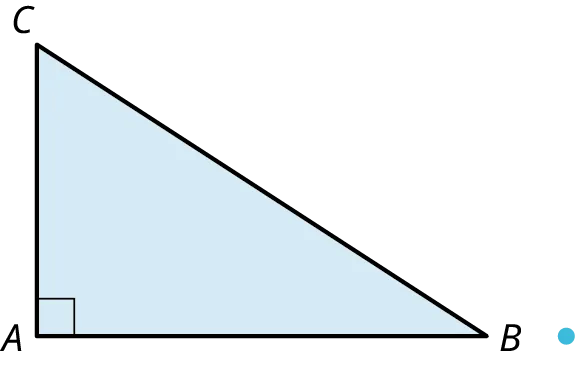11 .
Shapes can be rotated around a point of rotation or a ____________.
12 .
What is the name of the transformation that involves a reflection and a translation?
13 .
What can a tessellation not have between shapes?
14 .
Describe the transformation shown.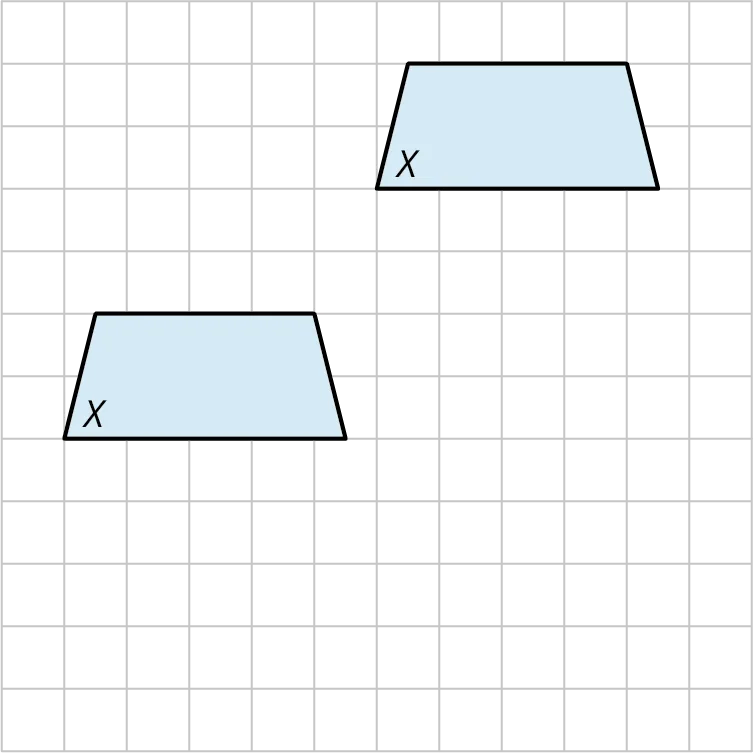15 .
What do we call a transformation that produces a mirror image?
16 .
Sketch the reflection of the shape about the dashed line.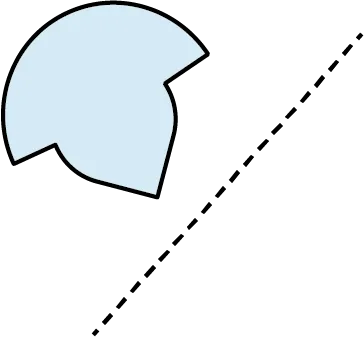17 .
Sketch the reflection of the shape about the dashed line.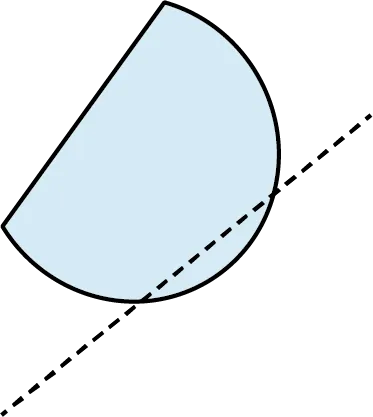18 .
Sketch the translation of the shape 3 units to the right and 3 units vertically.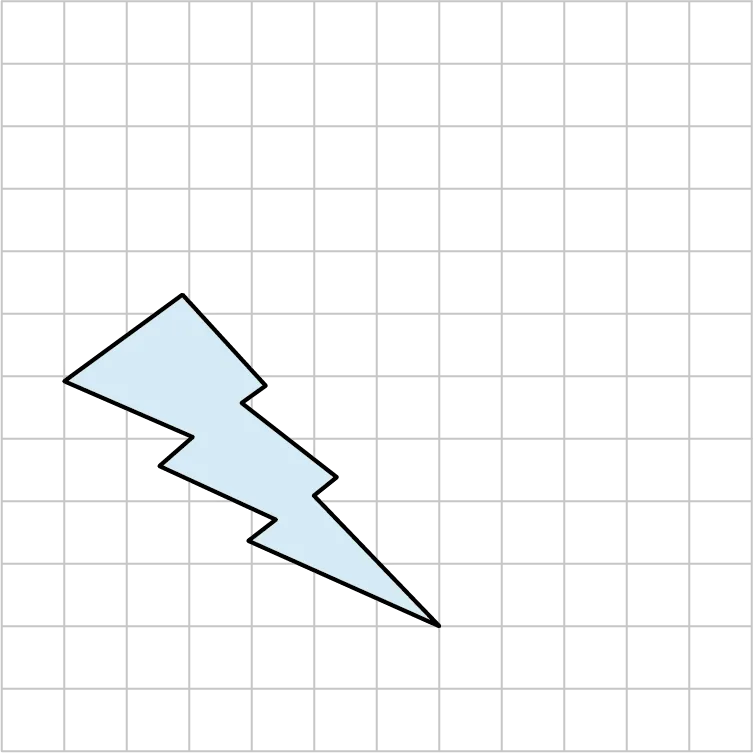19 .
Rotate the shape ${45^ \circ }$ about the rotation point using point $A$ as your guide.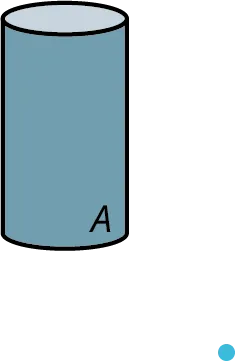20 .
Do regular pentagons tessellate the plain by themselves?
21 .
What do regular tessellations have in common?
22 .
How would we name a tessellation of squares as shown in the figure?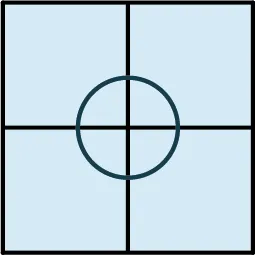23 .
How do we name a tessellation of octagons and squares as shown in the figure?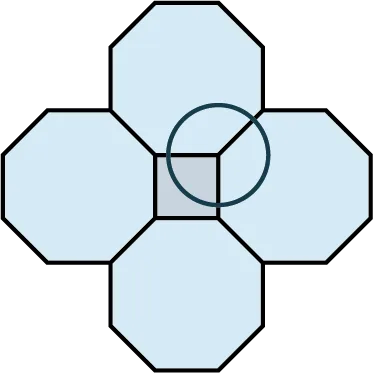24 .
How would we name a tessellation of trapezoids as shown in the figure?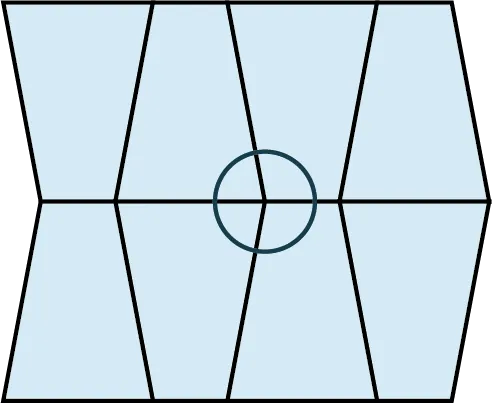Order a print copy

As an Amazon Associate we earn from qualifying purchases.